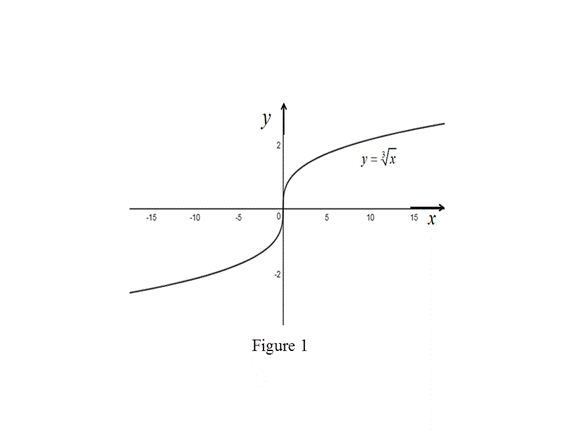# The derivative of the function f ( x ) at x = a if a ≠ 0 .### Single Variable Calculus: Concepts...

4th Edition
James Stewart
Publisher: Cengage Learning
ISBN: 9781337687805### Single Variable Calculus: Concepts...

4th Edition
James Stewart
Publisher: Cengage Learning
ISBN: 9781337687805

#### Solutions

Chapter 2.7, Problem 49E

(a)

To determine

## To find: The derivative of the function f(x) at x=a if a≠0.

Expert Solution

The value of f(a) is 13a23.

### Explanation of Solution

Given:

The given function is, f(x)=x3.

Result Used:

The derivative of a function f at x=a, denoted by f(x), is

f(a)=limxaf(x)f(a)xa (1)

Difference of cube formula: x3y3=(xa)(x2+y2+xy).

Calculation:

Obtain the derivative of the function f(x) at x=a.

Compute f(a) by using the equation (1),

f(a)=limxaf(x)f(a)xa=limxax3a3xa=limxa(x13a13)xa=limxa(x13a13)xa×(x23+a13x13+a23)(x23+a13x13+a23)

Apply the difference of cube formula in the numerator as follows,

f(a)=limxa(x13)3(a13)3xa×1(x23+a13x13+a23)=limxaxaxa×1(x23+a13x13+a23)=limxa1{x23+a13x13+a23}[ xa0]=limxa(1)limxa{x23+a13x13+a23}

=1a23+a13a13+a23

Simplify the denominator,

f(a)=1a23+a23+a23=13a23=13a23

Thus, the value of f(a) is 13a23.

(b)

To determine

Expert Solution

### Explanation of Solution

Result used:

The derivative of a function f , denoted by f(x), is

f(a)=limh0f(a+h)f(a)h (2)

Proof:

Consider the function f(x)=x3.

Compute f(0) by using the equation (2),

f(0)=limh00+h303h=limh0h30h=limh0h13h=limh01hh13

=limh01h23

Here, the function 1h23 becomes larger and larger as h tends to zero. That is,

f(0)=limh01h23=

Therefore, the derivative of the function does not exist at x=0.

Thus, the required proof is obtained.

(c)

To determine

Expert Solution

### Explanation of Solution

Result Used:

A curve has a vertical tangent line at x=a if f is continuous at x=a and limxa|f(x)|=

Proof:

Consider the equation f(x)=x3.

Substitute x=0 in f(x),

f(0)=03=0

Thus f(0)=0 is defined.

The limit of the function f(x) is computed as follows,

limx0f(x)=limx0x3=03=0

Therefore, f(x) is continuous at x=0.

From part (a), f(x)=x233

Take the limit of the function f(x) as x approaches zero.

limx0|f(x)|=limx0|13x23|=13limx0|1x23|=13limx01x23=[From part (b)]

Since the function f(x) is continuous at x=0 and limx0|f(x)|=.

By result, the curve f(x) has a vertical tangent at x=0.

Thus, the curve f(x) has a vertical tangent at the point (0,0).

Graph:

Use the online graphing calculator to draw the graph of the function y=x3 as shown below in Figure 1,From Figure 1, it is clear that the y-axis is the vertical tangent to the curve y=x3.

### Have a homework question?

Subscribe to bartleby learn! Ask subject matter experts 30 homework questions each month. Plus, you’ll have access to millions of step-by-step textbook answers!